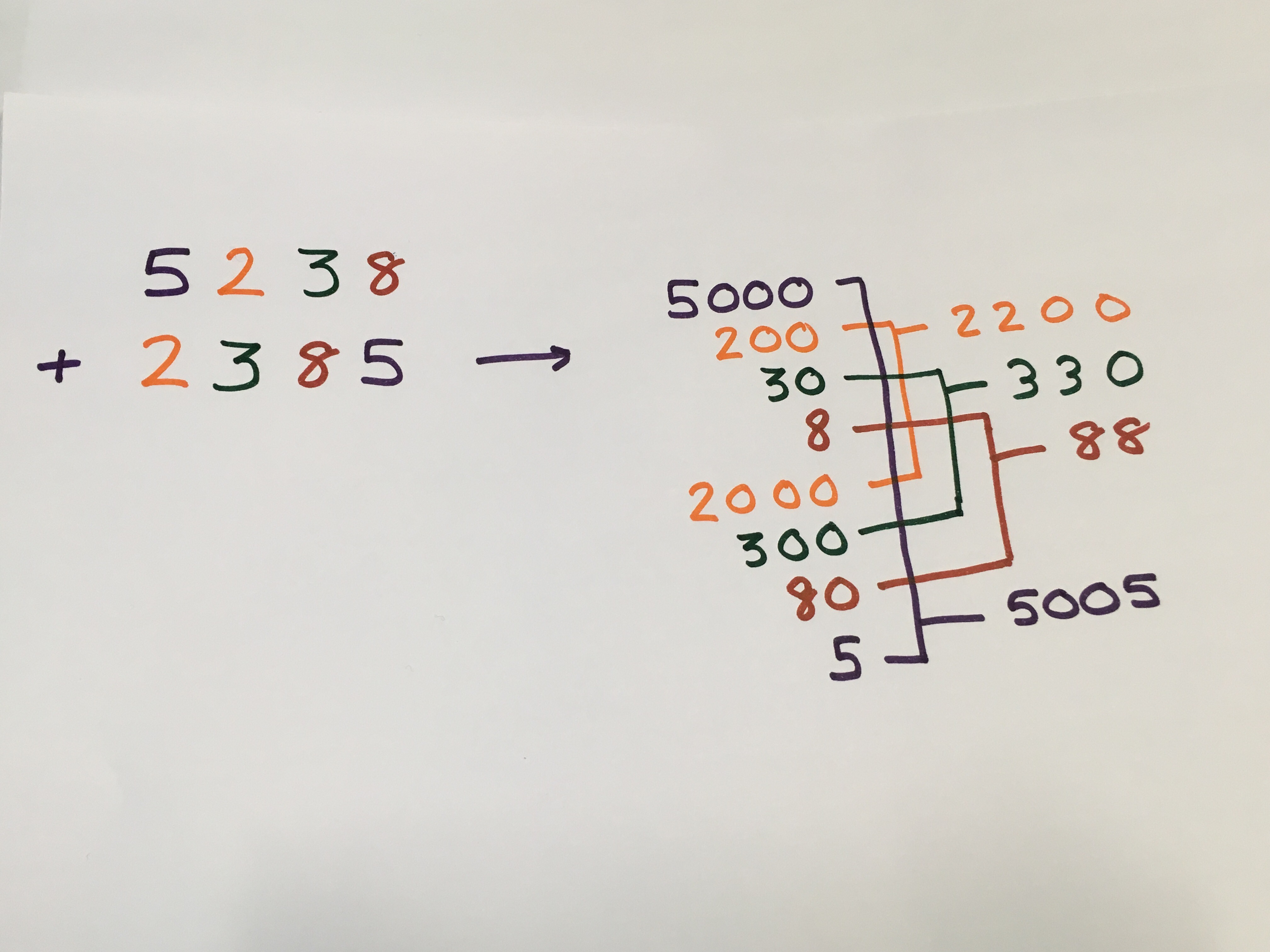#### You may also likeMake a set of numbers that use all the digits from 1 to 9, once and once only. Add them up. The result is divisible by 9. Add each of the digits in the new number. What is their sum? Now try some other possibilities for yourself!### Counting Factors

Is there an efficient way to work out how many factors a large number has?### Repeaters

Choose any 3 digits and make a 6 digit number by repeating the 3 digits in the same order (e.g. 594594). Explain why whatever digits you choose the number will always be divisible by 7, 11 and 13.

# Legs Eleven

##### Age 11 to 14Challenge Level

Imagine starting with the number $5238$.

We can write it as
$5000+200+30+8$
or
$5\times1000 + 2\times100 + 3\times10 + 8\times1$

Can you express the second number in the same way?

How many 5s, 2s, 3s and 8s are there when you combine the two numbers?